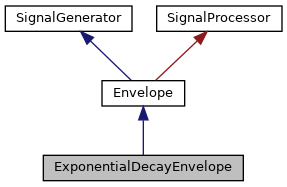OpenWareLaboratory
ExponentialDecayEnvelope Class Reference
Inheritance diagram for ExponentialDecayEnvelope:[legend]
Collaboration diagram for ExponentialDecayEnvelope:[legend]

## Public Member Functions

ExponentialDecayEnvelope (float sr)

virtual void gate (bool state)

virtual void gate (bool state, int gateDelay)

float getNextSample ()

void setDecay (float d)

void setRate (float r)

virtual void trigger (bool state)

virtual void trigger (bool state, int triggerDelay)

void trigger ()

## Static Public Member Functions

static ExponentialDecayEnvelopecreate (float sr)

static void destroy (ExponentialDecayEnvelope *env)

## Detailed Description

Definition at line 6 of file ExponentialDecayEnvelope.h.

## Constructor & Destructor Documentation

 ExponentialDecayEnvelope::ExponentialDecayEnvelope ( float sr )
inline

Definition at line 12 of file ExponentialDecayEnvelope.h.

Referenced by create().

## Member Function Documentation

 static ExponentialDecayEnvelope* ExponentialDecayEnvelope::create ( float sr )
inlinestatic

Definition at line 31 of file ExponentialDecayEnvelope.h.

References ExponentialDecayEnvelope().

 static void ExponentialDecayEnvelope::destroy ( ExponentialDecayEnvelope * env )
inlinestatic

Definition at line 34 of file ExponentialDecayEnvelope.h.

 virtual void Envelope::gate ( bool state )
inlinevirtualinherited

Definition at line 15 of file Envelope.h.

 virtual void Envelope::gate ( bool state, int gateDelay )
inlinevirtualinherited

Definition at line 18 of file Envelope.h.

 float ExponentialDecayEnvelope::getNextSample ( )
inline

Definition at line 26 of file ExponentialDecayEnvelope.h.

 void ExponentialDecayEnvelope::setDecay ( float d )
inline

Definition at line 20 of file ExponentialDecayEnvelope.h.

References setRate().

 void ExponentialDecayEnvelope::setRate ( float r )
inline

Definition at line 14 of file ExponentialDecayEnvelope.h.

Referenced by setDecay().

 virtual void Envelope::trigger ( bool state )
inlinevirtualinherited

Definition at line 11 of file Envelope.h.

References Envelope::trigger().

 virtual void Envelope::trigger ( bool state, int triggerDelay )
inlinevirtualinherited